• ## RMSprop

千次阅读 2018-12-05 16:40:28
RMSprop，全称：Root Mean Sqaure prop。 要看懂这篇博文，你需要先看懂： 指数加权平均 使用动量的梯度下降法 整理并翻译自吴恩达深度学习系列视频： ...ci...
RMSprop，全称：Root Mean Sqaure prop。
要看懂这篇博文，你需要先看懂：
指数加权平均使用动量的梯度下降法

整理并翻译自吴恩达深度学习系列视频： https://mooc.study.163.com/learn/2001281003?tid=2001391036#/learn/content?type=detail&id=2001702124&cid=2001693085

RMSprop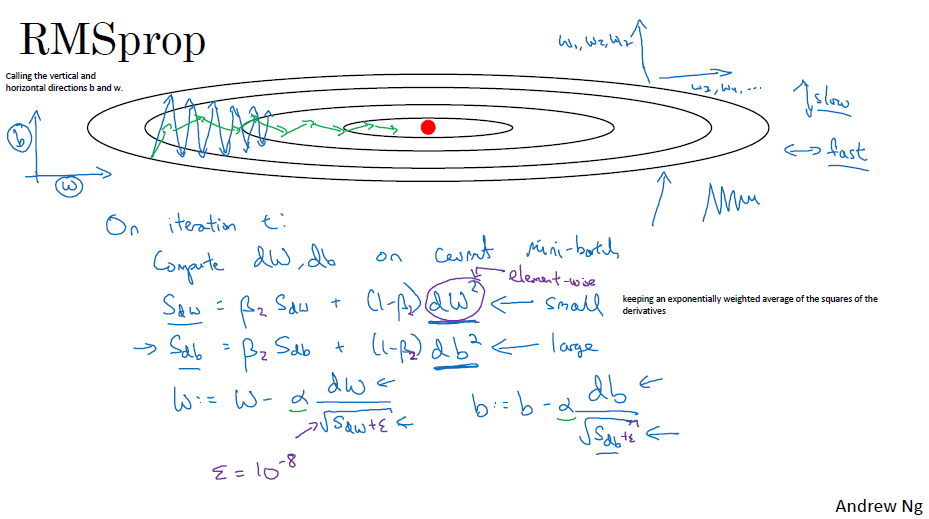同使用动量的梯度下降一样，RMSprop的目的也是为了消除垂直方向的抖动，使梯度下降快速收敛。
其计算方法(详见下)使用了将导数看作水平方向上的w和垂直方向上的b。并计算dw和db的平方值，这实际上是平方版本的指数加权平均。然后更新的时候又除去了根号求平均值，因此叫做Root Mean Sqaure prop。除法进行的时候因为dw较小，所以计算出的根号也较小，而db较大，所以计算出的根号也较大，因此更新时b变小了，w变化不大。
算法描述
该算法描述来自花书《deep learning》，与下面的计算方法不共享参数记号。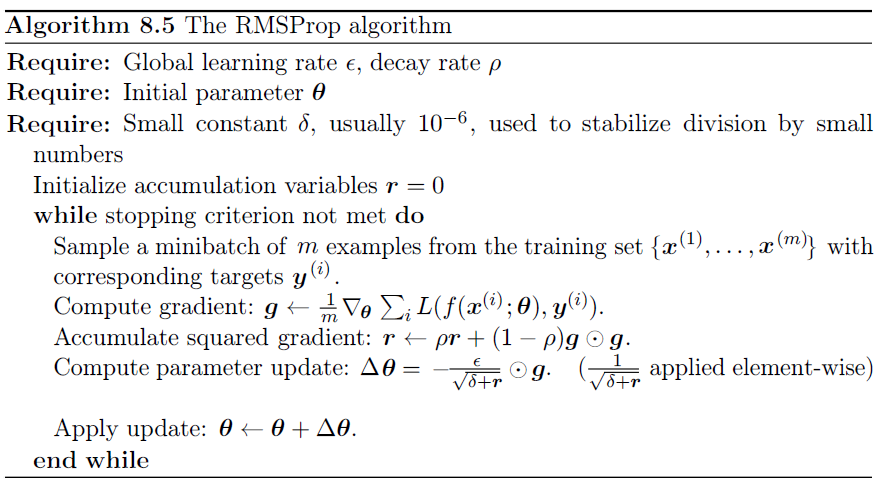RMSprop计算方法
在每一次迭代中，计算mini-batch上的：

S

d

w

=

β

2

S

d

w

+

(

1

−

β

2

)

d

W

2

S_{dw}=\beta_2S_{dw}+(1-\beta_2)dW^2

<- element-wise

S

d

b

=

β

2

S

d

b

+

(

1

−

β

2

)

d

b

2

S_{db}=\beta_2S_{db}+(1-\beta_2)db^2

<- element-wise
更新方法变成：

W

=

W

−

α

d

W

S

d

w

+

ϵ

W = W-\alpha \frac{dW}{\sqrt{S_{dw}+\epsilon}}

b

=

b

−

α

d

b

S

d

b

+

ϵ

b = b-\alpha \frac{db}{\sqrt{S_{db}+\epsilon}}

取

ϵ

=

1

0

−

8

\epsilon=10^{-8}

，目的是防止你除以0或者一个很小的数。
展开全文梯度下降
• RMSprop 这个算法的全称是root mean square prop.下面我们来谈一下他是如何工作的。 如图所示，你想减缓b方向的学习率，然后加速w方向的学习率。这里，所不同的是我们使用 Sdw=βSdw+(1−β)dw2Sdw=βSdw+(1...
RMSprop
这个算法的全称是root mean square prop.下面我们来谈一下他是如何工作的。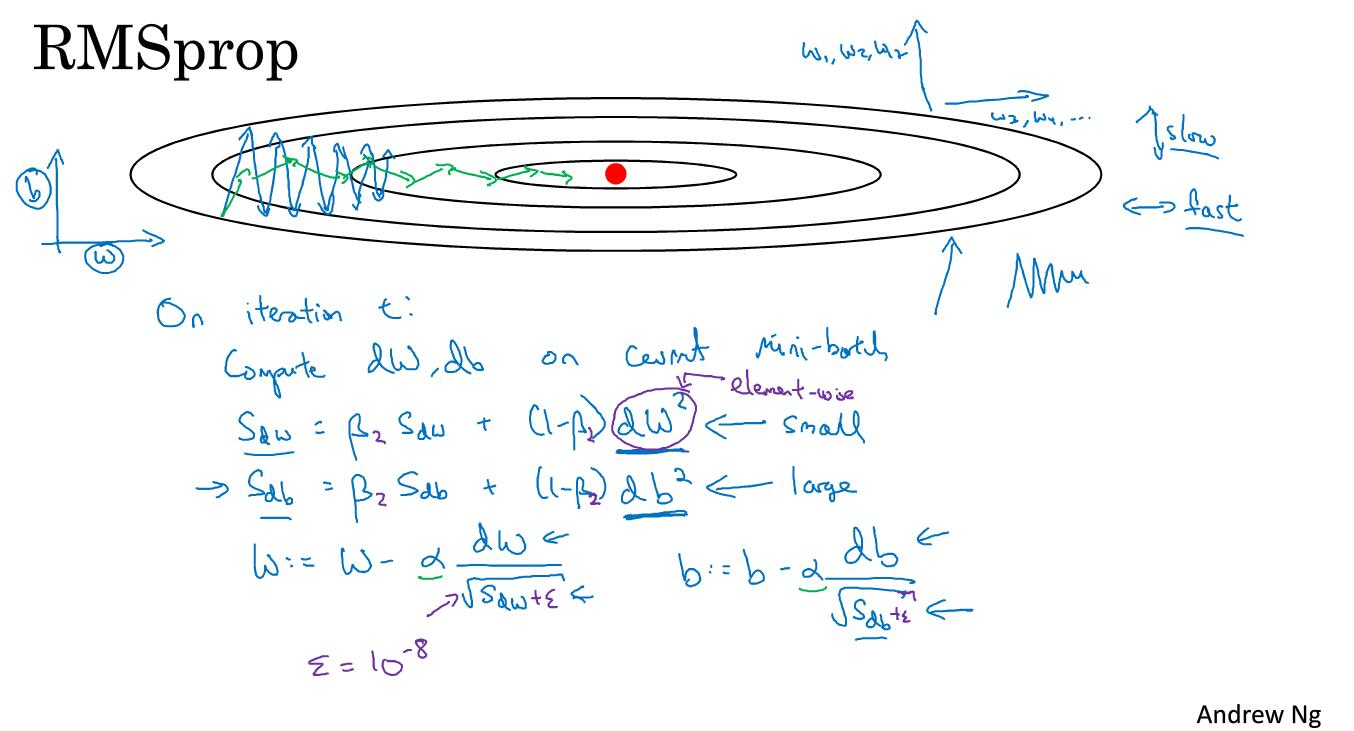如图所示，你想减缓b方向的学习率，然后加速w方向的学习率。这里，所不同的是我们使用

Sdw=βSdw+(1−β)dw2

S

d

w

=

β

S

d

w

+

(

1

−

β

)

d

w

2

S_{dw} = \beta S_{dw}+(1-\beta)dw^2

使用微分平方的加权平均数。

另外一个不同是最后w更新学习率的时候变得不同了。
我们来解释一下这个原理。我们希望dw要比较小，这样最后w的变化就会快。同时，我们也希望db要比较大，这样最后b的变化就不会那么快。我们从batch的变化图中的确可以看出，db变化是比较大的而dw相对比较小。因为在纵轴上，也就是b方向上函数倾斜程度更大一些。
所以最后RMSprop的变化情况就如同图中绿色线条的样子，这样，我们就可以选择更大的学习率，加快学习进度。
对于更高维的空间也是一样的道理，RMSporp的作用就是让那些抖动十分剧烈的部分变得平缓一些。
另外在实际情况中为了不让分母为0，所以我们加上一个十分十分小的数

ε

ε

$\varepsilon$，这个数具体是多少没有关系，它的作用是保证整体的稳定性。
展开全文RMSProp优化算法
参考：【优化算法】一文搞懂RMSProp优化算法再抛出原始的RMSProp算法:2.经验上，RMSProp被证明有效且实用的深度学习网络优化算法。直观理解作用
简单来讲，设置全局学习率之后，每次通过，全局学习率逐参数的除以经过衰减系数控制的历史梯度平方和的平方根，使得每个参数的学习率不同
那么它起到的作用是什么呢？
参数的学习率不同**
那么它起到的作用是什么呢？
起到的效果是在参数空间更为平缓的方向，会取得更大的进步（因为平缓，所以历史梯度平方和较小，对应学习下降的幅度较小），并且能够使得陡峭的方向变得平缓，从而加快训练速度。
展开全文神经网络 算法 深度学习 人工智能
• ## pytorch优化器详解：RMSProp

万次阅读 多人点赞 2020-09-10 18:19:24
RMSProp原理 假设损失函数是，即我们的目标是学习x和y的值，让Loss尽可能小。如下是绘制损失函数的代码以及绘制出的结果。注意这并不是一个U型槽，它有最小值点，这个点对应的x和y值就是学习的目标。 import nu
说明
模型每次反向传导都会给各个可学习参数p计算出一个偏导数，用于更新对应的参数p。通常偏导数不会直接作用到对应的可学习参数p上，而是通过优化器做一下处理，得到一个新的值，处理过程用函数F表示（不同的优化器对应的F的内容不同），即，然后和学习率lr一起用于更新可学习参数p，即。
RMSProp原理
假设损失函数是，即我们的目标是学习x和y的值，让Loss尽可能小。如下是绘制损失函数的代码以及绘制出的结果。注意这并不是一个U型槽，它有最小值点，这个点对应的x和y值就是学习的目标。
import numpy as np
from matplotlib import pyplot as plt
from mpl_toolkits.mplot3d import Axes3D

def func(x, y):
return x * x + 10 * y * y

def paint_loss_func():
x = np.linspace(-50, 50, 100) #x的绘制范围是-50到50，从改区间均匀取100个数
y = np.linspace(-50, 50, 100) #y的绘制范围是-50到50，从改区间均匀取100个数

X, Y = np.meshgrid(x, y)
Z = func(X, Y)

fig = plt.figure()#figsize=(10, 10))
ax = Axes3D(fig)
plt.xlabel('x')
plt.ylabel('y')

ax.plot_surface(X, Y, Z, rstride=1, cstride=1, cmap='rainbow')
plt.show()

paint_loss_func()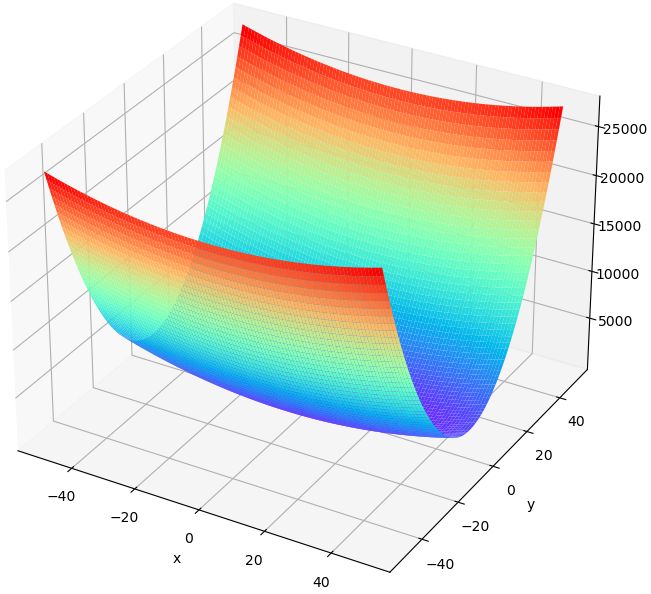通过解析求解，显然当时，Loss取得最小值。但这里我们通过神经网络反向传播求导的的方式，一步步优化参数，让Loss变小。通过这个过程，可以看出RMSProp算法的作用。
假设x和y的初值分别为，此时对Loss函数进行求导，x和y的梯度分别是，显然x将要移动的距离小于y将要移动的距离，但是实际上x离最优值0更远，差距是40，y离最优值0近一些，距离是20。因此SGD给出的结果并不理想。
RMSProp算法有效解决了这个问题。通过累计各个变量的梯度的平方r，然后用每个变量的梯度除以r，即可有效缓解变量间的梯度差异。如下伪代码是计算过程。
初始：（这里只有两个可学习参数）初始：学习率 初始：平滑常数（或者叫做衰减速率） 初始：，加在分母上防止除0初始：梯度的平方 （有几个参数就有几个r）while 没有停止训练 do        计算梯度：        累计梯度的平方：（也是一样的）        更新可学习参数：（y的更新也是一样的）end while
下图是训练10次，x和y的移动轨迹，其中红色对应SGD，蓝色对应RMSProp。观察SGD对应的红色轨迹，由于y的梯度很大，y方向移动过多，一下从坡一边跑到了另一边，而x的移动却十分缓慢。但是通过RMSProp，有效消除了梯度差异导致的抖动。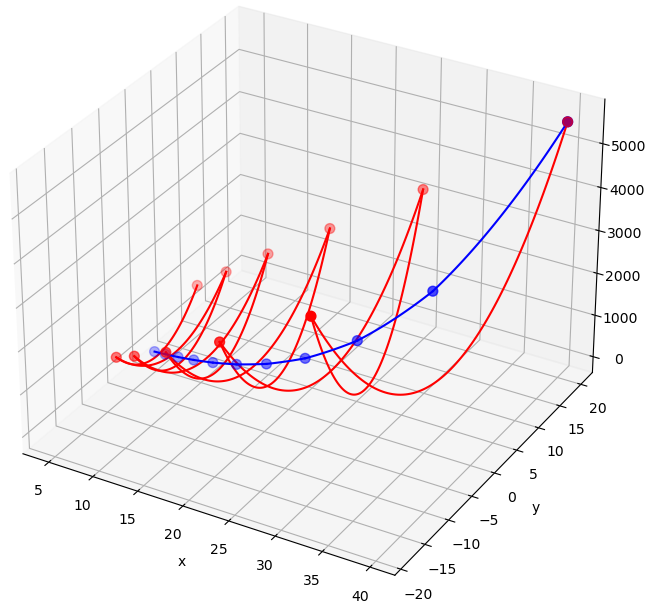训练过程的代码如下。
def grad(x, y): #根据上述代码，可知x和y的梯度分别为2x和20y
return 2 * x, 20 * y

def train_SGD():
cur_x = 40
cur_y = 20
lr = 0.096
track_x = [cur_x] #记录x每次的值
track_y = [cur_y] #记录y每次的值
for i in range(10): #作为demo，这里只训练10次
track_x.append(cur_x)
track_y.append(cur_y)
#print(track_x)
#print(track_y)
return track_x, track_y

def train_RMSProp():
cur_x = 40
cur_y = 20
lr = 3
r_x, r_y = 0, 0 #伪代码中的r
alpha = 0.9
eps = 1e-06
track_x = [cur_x]
track_y = [cur_y]
for i in range(10):
r_x = alpha * r_x + (1 - alpha) * (grad_x * grad_x)
r_y = alpha * r_y + (1 - alpha) * (grad_y * grad_y)
cur_x -= (grad_x / (np.sqrt(r_x) + eps)) * lr
cur_y -= (grad_y / (np.sqrt(r_y) + eps)) * lr
track_x.append(cur_x)
track_y.append(cur_y)
#print(track_x)
#print(track_y)
return track_x, track_y

def paint_tracks(track_x1, track_y1, track_x2, track_y2):
x = np.linspace(-50, 50, 100)
y = np.linspace(-50, 50, 100)

X, Y = np.meshgrid(x, y)
Z = func(X, Y)

fig = plt.figure(figsize=(10, 10))
ax = Axes3D(fig)
plt.xlabel('x')
plt.ylabel('y')

#ax.plot(track_x, track_y, func(np.array(track_x), np.array(track_y)), 'r--')
tx1, ty1 = track_x1, track_y1
for i in range(1, len(track_x1)):
tx2, ty2 = track_x1[i], track_y1[i]
tx = np.linspace(tx1, tx2, 100)
ty = np.linspace(ty1, ty2, 100)
ax.plot(tx, ty, func(tx, ty), 'r-')
tx1, ty1 = tx2, ty2
ax.scatter(track_x1, track_y1, func(np.array(track_x1), np.array(track_y1)), s=50, c='r')

tx1, ty1 = track_x2, track_y2
for i in range(1, len(track_x2)):
tx2, ty2 = track_x2[i], track_y2[i]
tx = np.linspace(tx1, tx2, 100)
ty = np.linspace(ty1, ty2, 100)
ax.plot(tx, ty, func(tx, ty), 'b-')
tx1, ty1 = tx2, ty2
ax.scatter(track_x2, track_y2, func(np.array(track_x2), np.array(track_y2)), s=50, c='b')
#ax.plot_surface(X, Y, Z, rstride=1, cstride=1, cmap='rainbow')
plt.show()

track_x_sgd, track_y_sgd = train_SGD()
#paint_track(track_x_sgd, track_y_sgd)

track_x_rms, track_y_rms = train_RMSProp()
paint_tracks(track_x_sgd, track_y_sgd, track_x_rms, track_y_rms)
pytorch RMSProp参数
接下来看下pytorch中的RMSProp优化器，函数原型如下，其中最后三个参数和RMSProp并无直接关系。
torch.optim.RMSprop(params,
lr=0.01,
alpha=0.99,
eps=1e-08,
weight_decay=0,
momentum=0,
centered=False)
params
模型里需要被更新的可学习参数，即上文的x和y。
lr
学习率。
alpha
平滑常数。
eps
，加在分母上防止除0
weight_decay
weight_decay的作用是用当前可学习参数p的值修改偏导数，即：，这里待更新的可学习参数p的偏导数就是。
weight_decay的作用是正则化，和RMSProp并无直接关系。
momentum
根据上文伪代码第8行，计算出后，如果，则继续后面的计算，即。
否则计算过程变成，其中初始为0，是x的梯度，是上述累计的x的梯度的平方。
momentum和RMSProp并无直接关系。
centered
如果centerd为False，则按照上述伪代码计算，即分母是。
否则计算过程变成，这里初始为0，然后分母依然是，但是不一样了。
centered和RMSProp并无直接关系，是为了让结果更平稳。
展开全文深度学习 pytorch神经网络优化算法 momentum Adagrad Adam
• 类似于Momentum,是通过消除梯度下降过程中的摆动来加速下降的方法。 不同于Momentum类似，他是从...optimizer = keras.optimizers.RMSprop(0.01) 0.01是超参数，可以调整。其所在位置就是原来学习率所在的位置。 ...人工智能 深度学习 tensorflow深度学习 机器学习 神经网络 人工智能 算法优化算法 神经网络
• ## 模型优化-RMSprop

万次阅读 2019-06-02 22:54:41
RMSprop 全称 root mean square prop 算法，和动量方法一样都可以加快梯度下降速度。关于动量方法的内容可以参考这篇博文模型优化-动量方法。 动量方法借助前一时刻的动量，从而能够有效地缓解山谷震荡以及鞍部停滞...模型优化
• RMSprop 知道了动量（ Momentum）可以加快梯度下降，还有一个叫做 RMSprop 的算法，全称是 root mean square prop 算法，它也可以加速梯度下降： 如果你执行梯度下降，虽然横轴方向正在推进，但纵轴方向会有大...深度学习 机器学习 神经网络 优化算法
• tensorflow中RMSprop优化器运用 RMSprop优化器引用API：tf.keras.optimizers.RMSprop 代码实现： #RMSprop beta = 0.9 #定义超参数，经验值为0.9 v_w = beta * v_w + (1 - beta) * tf.square...神经网络 python 深度学习 tensorflow 机器学习
• RMSprop梯度下降法 有点类似动量梯度下降法，其过程为： 我们在神经网络中，算出了dw,dbdw,dbdw,db，然后令： Sdw:=βSdw+(1−β)dw2Sdb:=βSdb+(1−β)db2  w:=w−α⋅dwSdw+10−8b:=b−α⋅dbSdb+10−8Sdw:=\...机器学习 自然语言处理
• Pytorch Note18 优化算法4 RMSprop算法 文章目录Pytorch Note18 优化算法4 RMSprop算法RMSpropRMSProp 算法代码从0实现pytorch内置优化器可视化 全部笔记的汇总贴：Pytorch Note 快乐星球 RMSprop RMSprop 是由 ...深度学习 python 神经网络 机器学习 pytorch
• RMSProp RMSProp通过引入一个衰减系数，让r每回合都衰减一定比例，类似于Momentum中的做法。 具体实现:  需要:全局学习速率 ϵ, 初始参数 θ, 数值稳定量δ，衰减速率ρ 中间变量: 梯度累计量r(初始化为0)  每...
• ## pytorch学习笔记（三十七）：RMSProp

千次阅读 多人点赞 2020-08-10 13:06:45深度学习 人工智能 机器学习 pytorch
• ## Deep Learning 最优化方法之RMSProp

万次阅读 多人点赞 2017-05-21 23:02:50深度学习 优化
• 要说Momentum、RMSprop、Adam这三个优化器就要先讲讲指数加权平均 指数加权平均 上图是一年中365天的温度变化 指数加权平均的等式是：Vt = βVt-1 + (1-β)θt θt 代表第t天的温度，也就是纵轴的数值，那么这个...深度学习
• rmsprop(lr, tparams, grads, x, mask, y, cost):  """  A variant of SGD that scales the step size by running average of the  recent step norms.自适应梯度深度学习 算法...